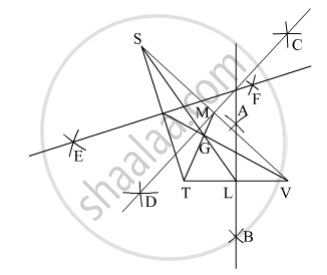SSC (English Medium) Class 8Maharashtra State Board
Share

# Draw an Obtuse Angled δ Stv. Draw Its Medians and Show the Centroid. - SSC (English Medium) Class 8 - Mathematics

ConceptMedians of a Triangle

#### Question

Draw an obtuse angled Δ STV. Draw its medians and show the centroid.

#### SolutionSteps of construction :
(i) Draw an obtuse angled ∆STV.
(ii) Draw the perpendicular bisector AB of side TV that intersect side TV at L. L is the mid point of TV.
(iii) Join SL, where SL is median to the side TV.
In the same manner, obtain the mid points M and N of sides SV and ST respectively.
(iv) Join TM and VN.
Hence, ∆STV is the required triangle in which the medians SL, TM and VN to the sides TV, SV and ST respectively intersect at point G.
The point G is the centroid of ∆STV.

Is there an error in this question or solution?

#### APPEARS IN

Balbharati Solution for Balbharati Class 8 Mathematics (2019 to Current)
Chapter 4: Altitudes and Medians of a triangle
Practice Set 4.1 | Q: 3 | Page no. 22
Solution Draw an Obtuse Angled δ Stv. Draw Its Medians and Show the Centroid. Concept: Medians of a Triangle.
S